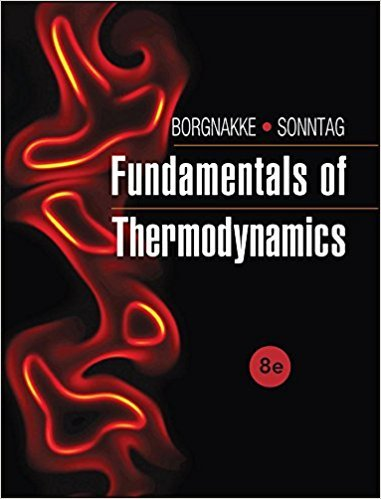×
Get Full Access to Fundamentals Of Thermodynamcs - 8 Edition - Chapter 2 - Problem 61hp
Get Full Access to Fundamentals Of Thermodynamcs - 8 Edition - Chapter 2 - Problem 61hp

×

# A boiler feed pump delivers 0.05 m3/s of water at 240°C,ISBN: 9781118131992 58

## Solution for problem 61HP Chapter 2

Fundamentals of Thermodynamcs | 8th Edition

• Textbook Solutions
• 2901 Step-by-step solutions solved by professors and subject experts
• Get 24/7 help from StudySoup virtual teaching assistantsFundamentals of Thermodynamcs | 8th Edition

4 5 1 405 Reviews
24
0
Problem 61HP

Problem 61HP

A boiler feed pump delivers 0.05 m3/s of water at 240°C, 20 MPa. What is the mass flow rate (kg/s)? What would be the percent error if the properties of saturated liquid at 240°C were used in the calculation? What if the properties of saturated liquid at 20 MPa were used?

Step-by-Step Solution:

Solution 61 HP

Step 1 of 4

We are required to calculate the mass flow rate

We need to calculate the percentage error if the properties of saturated liquid at 240°C.

We need to calculate the percentage error if the properties of saturated liquid at 20 MPa.

Step 2 of 4

Step 3 of 4

##### ISBN: 9781118131992

The full step-by-step solution to problem: 61HP from chapter: 2 was answered by , our top Engineering and Tech solution expert on 08/03/17, 05:05AM. This full solution covers the following key subjects: were, used, saturated, properties, liquid. This expansive textbook survival guide covers 7 chapters, and 1462 solutions. This textbook survival guide was created for the textbook: Fundamentals of Thermodynamcs , edition: 8. Since the solution to 61HP from 2 chapter was answered, more than 395 students have viewed the full step-by-step answer. The answer to “A boiler feed pump delivers 0.05 m3/s of water at 240°C, 20 MPa. What is the mass flow rate (kg/s)? What would be the percent error if the properties of saturated liquid at 240°C were used in the calculation? What if the properties of saturated liquid at 20 MPa were used?” is broken down into a number of easy to follow steps, and 51 words. Fundamentals of Thermodynamcs was written by and is associated to the ISBN: 9781118131992.

Unlock Textbook Solution